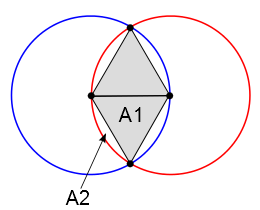#### You may also like### Baby Circle

A small circle fits between two touching circles so that all three circles touch each other and have a common tangent? What is the exact radius of the smallest circle?### Kissing

Two perpendicular lines are tangential to two identical circles that touch. What is the largest circle that can be placed in between the two lines and the two circles and how would you construct it?### Logosquares

Ten squares form regular rings either with adjacent or opposite vertices touching. Calculate the inner and outer radii of the rings that surround the squares.

# 2D-3D

##### Age 16 to 18Challenge Level

Well done Daniel Tuck for your solution and also Tony Cardell, age 14, State College Area High School, Pennsylvania, USA

The first part requires only some very elementary geometry and knowledge of how to work out the area of circles and triangles. The solution given here uses calculus for finding the volume in the second part. If you can find this volume without calculus we'll be pleased to publish your method.We may say that the distance between the two centers of the circles is $r$ (since each lies on the other circumference). Notice also that the intersection points of the two circles are also $r$ away from both centers (by definition of being the intersection of equal size circles with radius $r$). Thus the two centers and any one of the two intersection points forms an equilateral triangle of side $r$. The area of each equilateral triangle is

$A_1 = {r \over2}\times{r\sqrt 3 \over 2}$ that is

$A_1 = {r^2\sqrt3 \over 4}.$

So the area of the region is equal to twice the area of an equilateral triangle with side $r$ plus the extra outside the triangle. Since an equilateral triangle has angles of 60 degrees, one sixth of 360, the number of degree in a circle, the area of this extra region is 1/6 the area of a circle minus the area of an equilateral with sides $r$.

$A_2 = {\pi r^2 \over 6} - {r^2\sqrt3 \over 4}.$

There are 4 of these extra regions, so our total area of the region in $2D$ is

\eqalign { 4A_2 + 2A_1 &= 4\left({\pi r^2 \over 6} - {r^2\sqrt3 \over 4}\right) + 2\left({r^2\sqrt3 \over 4}\right) \cr &= {2\pi r^2 \over 3} - {r^2 \sqrt3 \over 2} .}

The volume between two spheres in a similar position is slightly more complex. To make the drawing of the circles into one of spheres, say the $x$-axis is a line through the diameters from left to right of the circles. Rotate the circles around the $x$-axis and you will sweep out a similar drawing with spheres.

Taking the origin O of the coordinate system at one of the centers then the points $P_1$ and $P_2$ of intersection of the circles drawn in 2D are at

$({r\over 2}, \pm{r\sqrt3 \over 2}).$

Consider the arc joining the origin to the point $P_1$. This is an arc of the circle center $(r,0)$ radius $r$ which has equation: $(x-r)^2 + y^2 = r^2$

or

$y = \sqrt(2xr - x^2).$

The volume of the intersection of the spheres is double the volume of revolution formed when this arc is rotated through 360 degrees about the $x$ axis.

\eqalign { \rm{Volume} &= 2\int_0^{r/2}\pi (2xr - x^2)dx \cr &= 2\pi \left[x^2r - {x^3 \over 3}\right]_0^{r/2} \cr &= 2\pi \left[{r^3\over 4} - {r^3 \over 24}\right] \cr &= {5\pi r^3 \over 12}. }